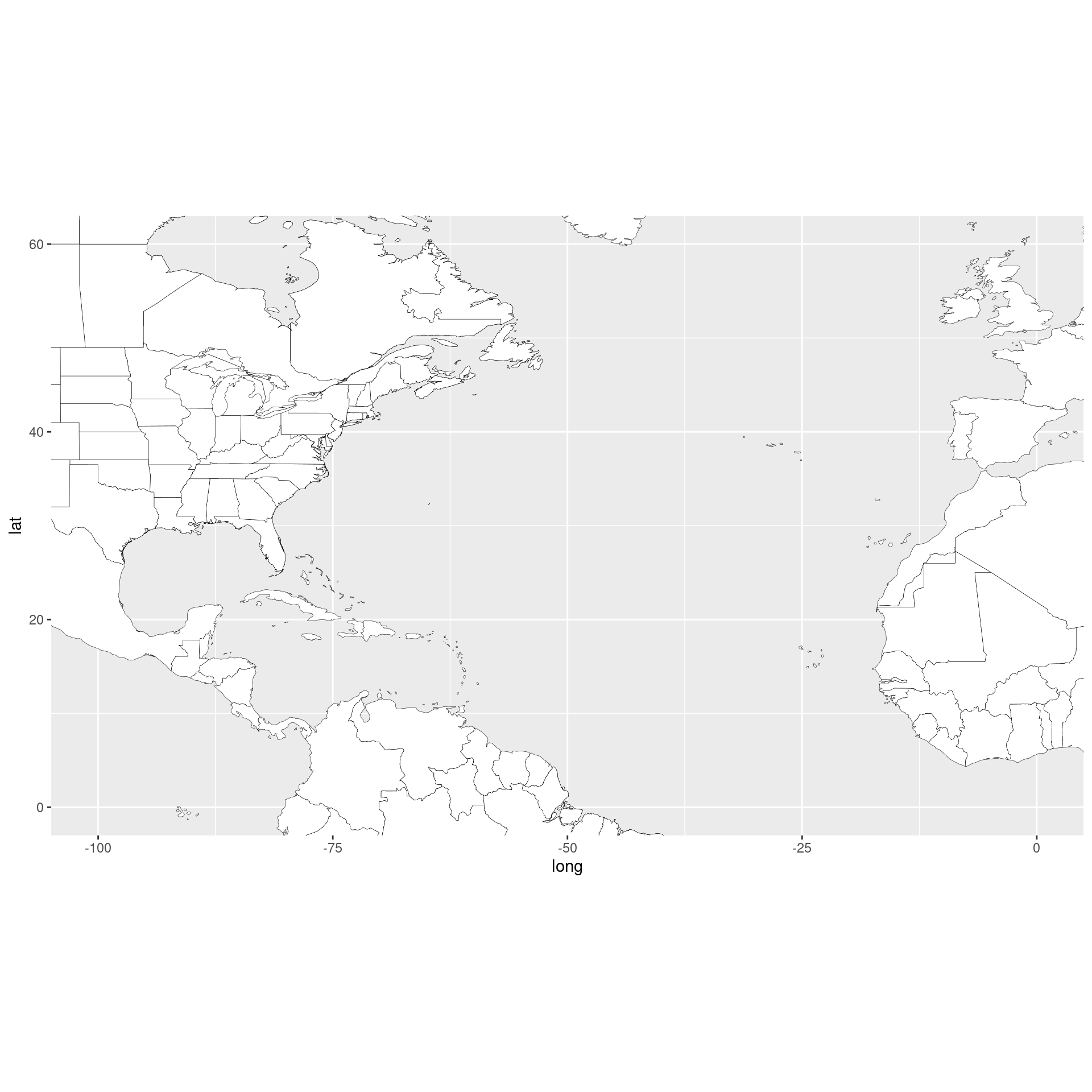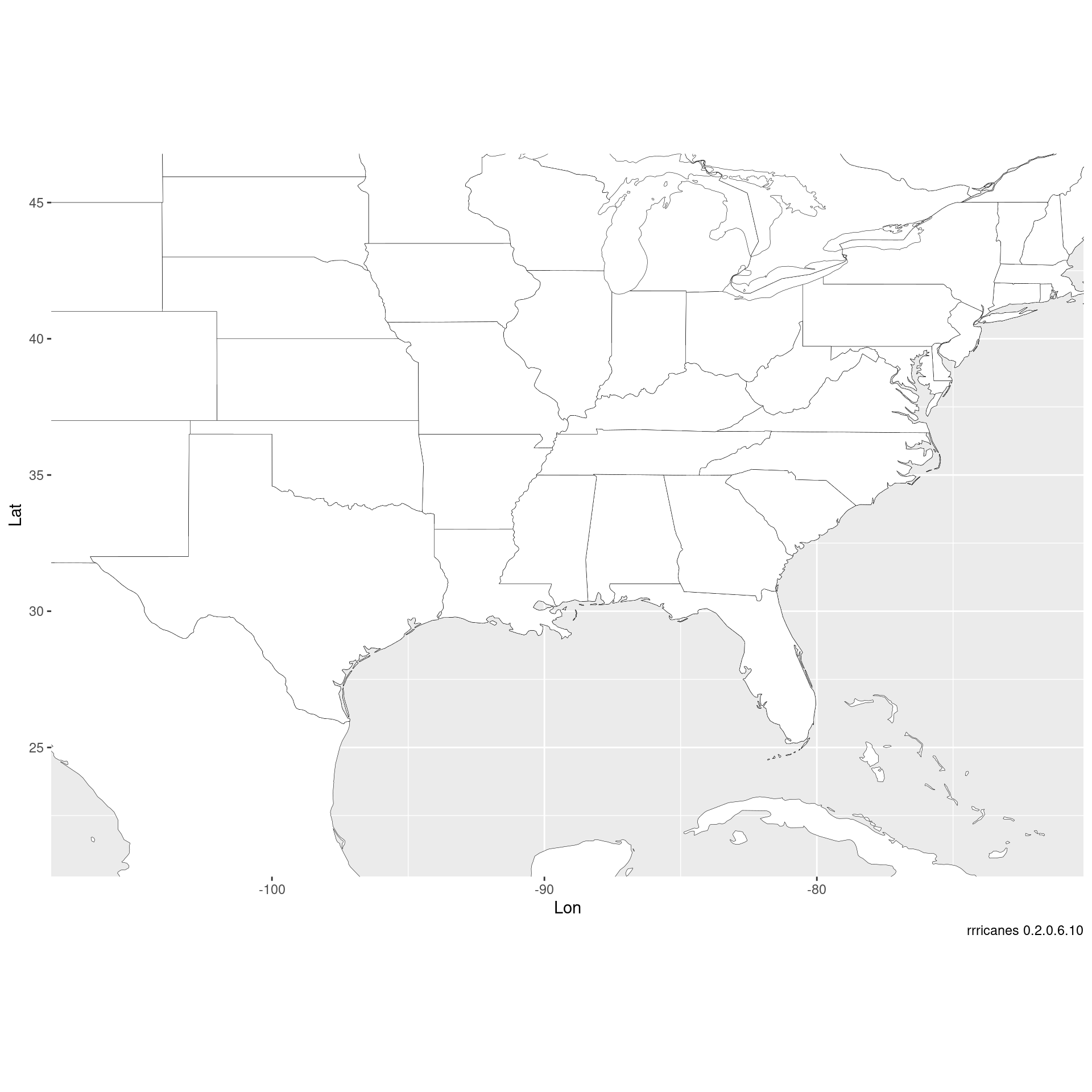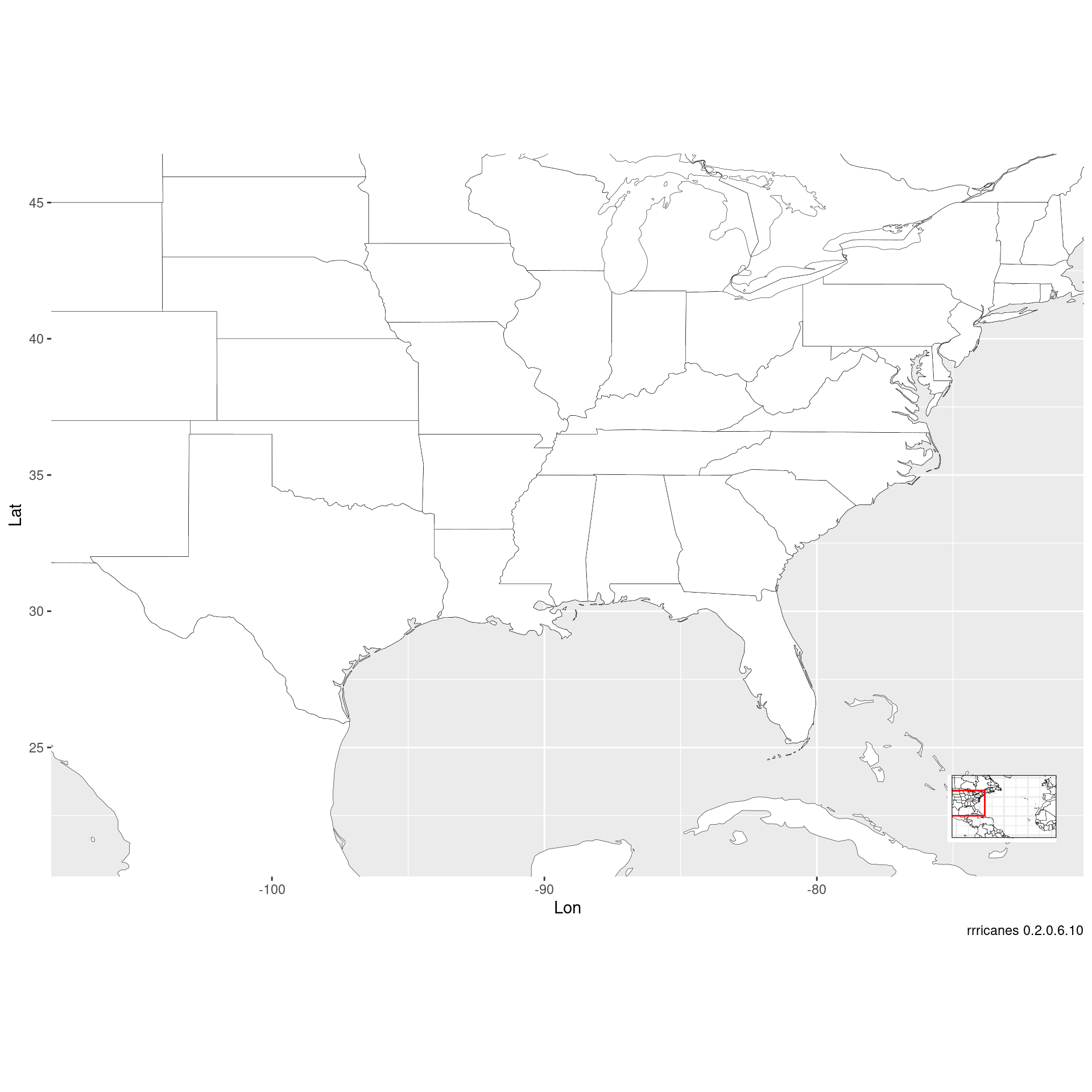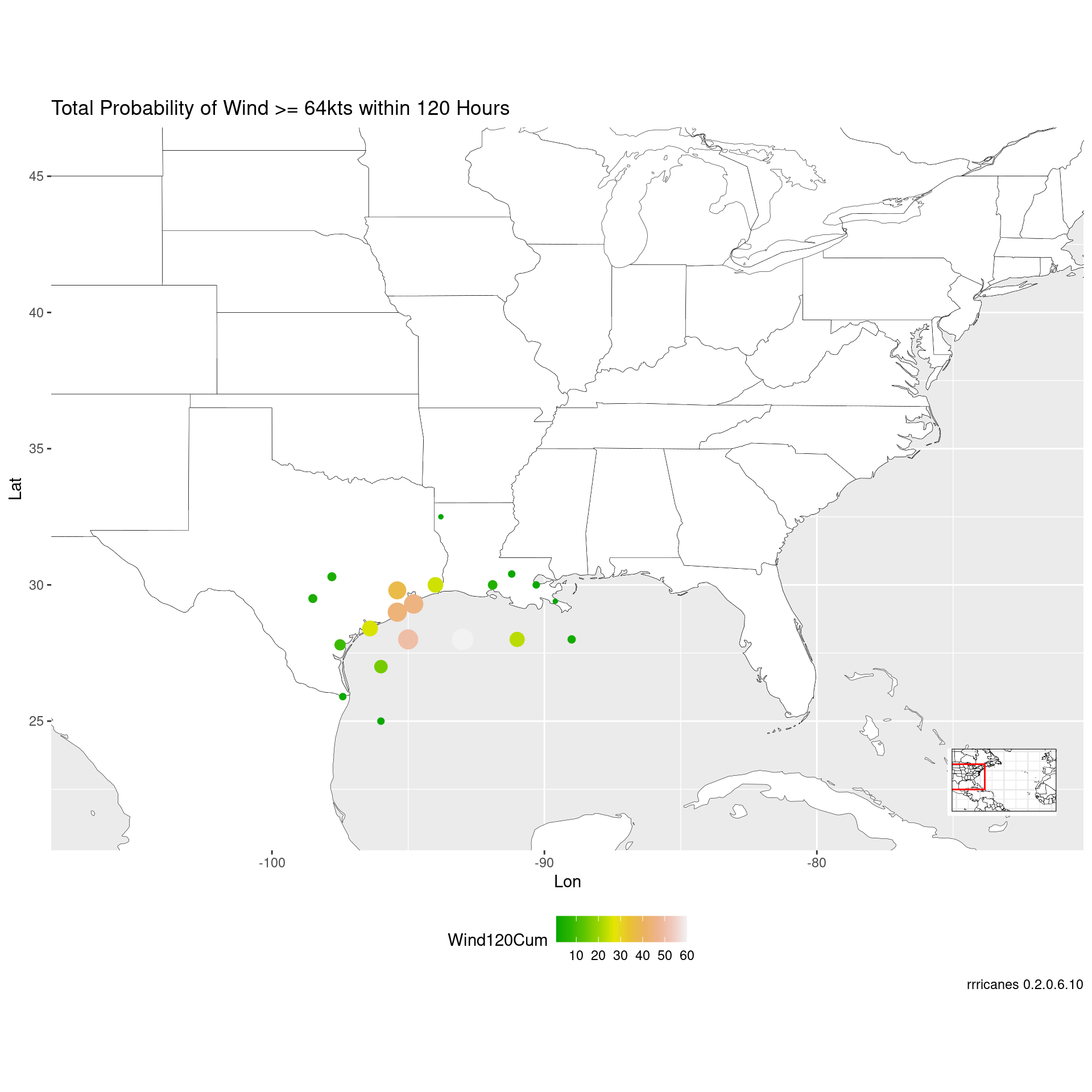``````library(dplyr)
library(ggplot2)
library(rrricanes)
library(rrricanesdata)
library(sp)``````
``````key <- "AL092008"
``wndprb <- wndprb %>% filter(Key == key, Adv <= adv)``

### GIS Advisory Forecast Track, Cone of Uncertainty, and Watches/Warnings

``gis_adv <- gis_advisory(key = key, advisory = adv) %>% gis_download()``
``````## OGR data source with driver: ESRI Shapefile
## Source: "/tmp/RtmprLdDmQ", layer: "al092008.042_5day_lin"
## with 2 features
## It has 9 fields
## OGR data source with driver: ESRI Shapefile
## Source: "/tmp/RtmprLdDmQ", layer: "al092008.042_5day_pgn"
## with 2 features
## It has 9 fields
## OGR data source with driver: ESRI Shapefile
## Source: "/tmp/RtmprLdDmQ", layer: "al092008.042_5day_pts"
## with 13 features
## It has 20 fields
## OGR data source with driver: ESRI Shapefile
## Source: "/tmp/RtmprLdDmQ", layer: "al092008.042_ww_wwlin"
## with 5 features
## It has 10 fields``````

Get bounding box of the forecast polygon.

``````bbox <- bbox(gis_adv\$al092008.042_5day_pgn)
bbox``````
``````##         min       max
## x -98.10178 -80.20625
## y  25.25667  41.78703``````

## Build a Tracking Chart

Generate a base plot of the Atlantic ocean.

``bp <- al_tracking_chart(color = "black", fill = "white", size = 0.1, res = 50)``
``````## Regions defined for each Polygons
## Regions defined for each Polygons``````
``## Coordinate system already present. Adding new coordinate system, which will replace the existing one.``
``bp``I like to add a little cushion for the map inset and forecast cone data.

``````lat_min <- bbox[2,1] - 5
lat_max <- bbox[2,2] + 5
lon_min <- bbox[1,1] - 10
lon_max <- bbox[1,2] + 10``````

Build a thin tracking map for the inset.

``````bp_inset <- ggplotGrob(bp +
geom_rect(mapping = aes(xmin = lon_min, xmax = lon_max,
ymin = lat_min, ymax = lat_max),
color = "red", alpha = 0) +
theme_bw() +
theme(axis.title = element_blank(),
axis.ticks = element_blank(),
axis.text.x = element_blank(),
axis.text.y = element_blank(),
plot.margin = margin(0, 0, 0, 0, "pt")))``````

Modify original `bp` zoomed in on our area of interest.

``````bp <- bp +
coord_equal(xlim = c(lon_min, lon_max),
ylim = c(lat_min, lat_max)) +
scale_x_continuous(expand = c(0, 0)) +
scale_y_continuous(expand = c(0, 0)) +
labs(x = "Lon",
y = "Lat",
caption = sprintf("rrricanes %s", packageVersion("rrricanes")))``````
``## Coordinate system already present. Adding new coordinate system, which will replace the existing one.``
``bp``Combine `bp` and `bp_inset` to finalize initial base plot. `bp` will be a base plot without the inset. `bpi` will have the inset.

``````bpi <- bp + annotation_custom(grob = bp_inset, xmin = lon_max - 5,
xmax = lon_max - 1, ymin = -Inf,
ymax = lat_min + 5)
bpi``````The `wndprb` will not have coordinates for cities. An option is `al_prblty_stations`. However, please note this function may become deprecated.

``````wndprb <-
wndprb %>%
left_join(al_prblty_stations(), by = "Location") %>%
mutate_at(.vars = c("Lat", "Lon"), .funs = as.numeric)``````
``## Warning: Expected 7 pieces. Additional pieces discarded in 1 rows .``

Check `wndprb` for NA values in `Lat`, `Lon`.

``any(is.na(wndprb\$Lat), is.na(wndprb\$Lon))``
``##  TRUE``
``````wndprb_adv42 <- filter(wndprb, Adv == adv, Wind >= 64)

bpi +
geom_point(
aes(
x = Lon,
y = Lat,
color = Wind120Cum,
size = Wind120Cum
)
) +